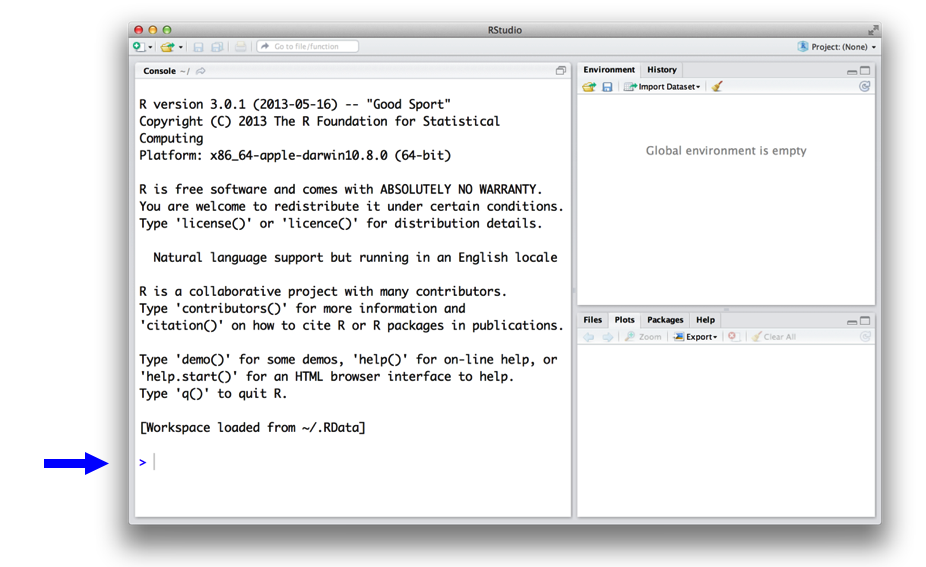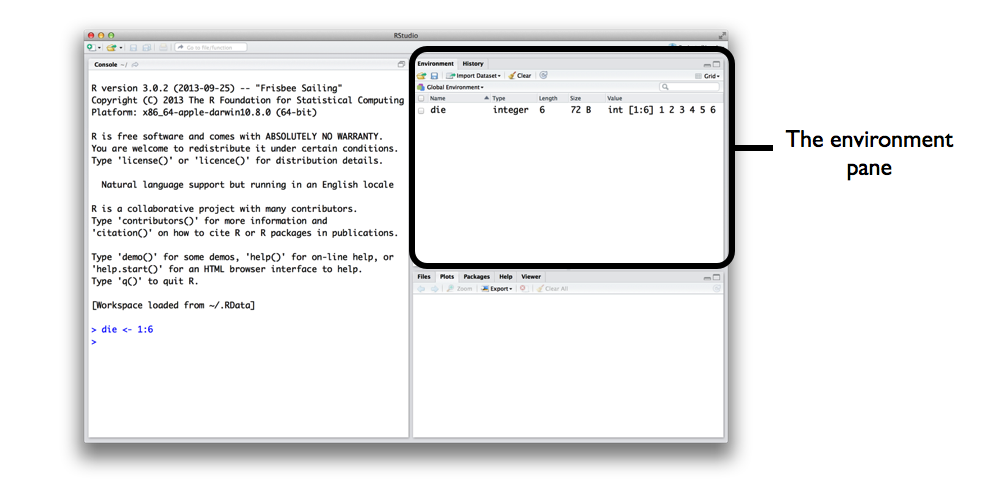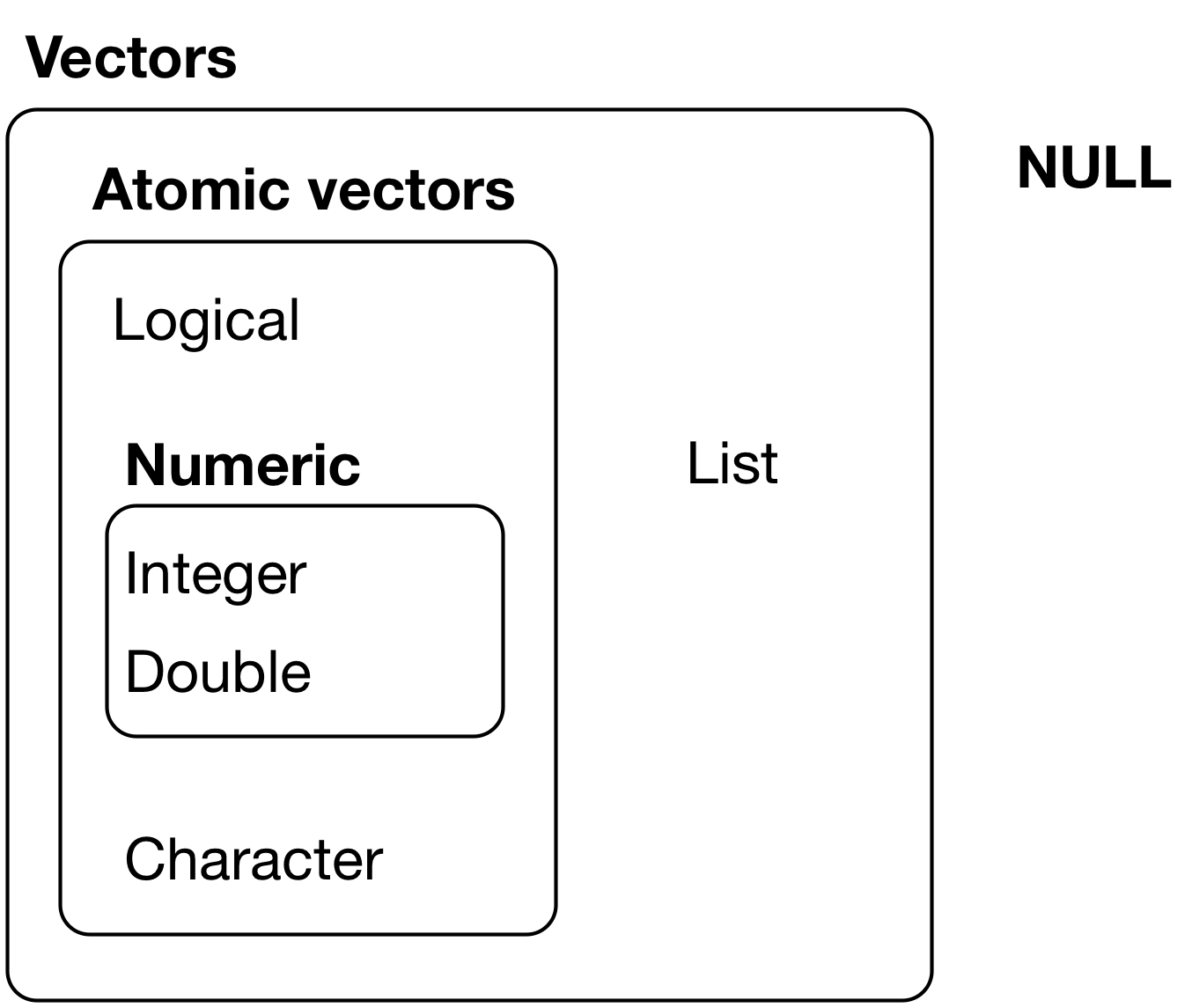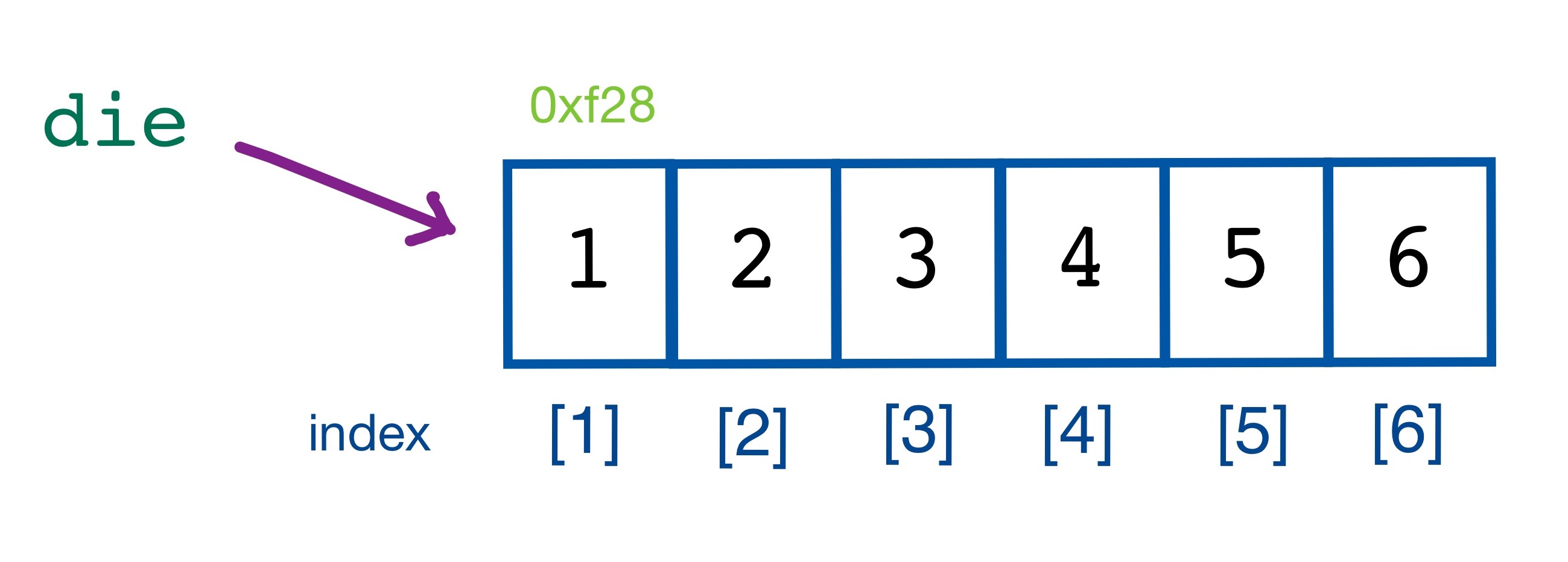## Agenda

• The R User Interface
• Command Line Interface
• Objects/Variables
• Atomic Vector
• Built-in Functions

## The R User Interface## Interacting with R

• You can use R interactively through its command line interface (CLI) or console:
• enter a command
• R executes the command
• get a printed response
• (repeat)
• You can use R in batch mode:
• give R a script: a list of commands
• R executes the commands
• get result(s) displayed on screen or stored in files: text, tables, graphics, and more

## Command Line Interface

`1 + 1`
`##  2`
`4 * 1.3`
`##  5.2`
`4 / 7`
`##  0.5714286`

## Command Line Interface

`4 / 7`
`##  0.5714286`
• ``: this line begins with the first value in the result.
`50:89`
```##   50 51 52 53 54 55 56 57 58 59 60 61 62 63 64 65 66 67 68 69 70 71 72 73 74
##  75 76 77 78 79 80 81 82 83 84 85 86 87 88 89```
• `:` returns every integer between two integers.
• The result returned is of type vector.

## Live Coding Exercise!

• Choose any number and add 2 to it.
• Multiply the result by 3.
• Subtract 6 from the answer.
• Divide what you get by 3.

Let’s code!

## R Objects/Variables

• Object/variable is a name that you can use to call up stored data.
• To assign/store value to an object/variable, use `=` or `<-` notation.
```a <- 1
a```
`##  1`
`a + 2`
`##  3`

## R Objects/Variables

```die <- 1:6
die```
`##  1 2 3 4 5 6`## R Objects/Variables

Good names Names that cause errors
a 1trial
b \$
FOO ^mean
my_var 2nd
.day !bad
• R is case-sensitive.
• `name` and `Name` will refer to different objects.

## R Vector

• In R, vector includes: atomic vector and list.## R Vector

• Vector stores its values as a one-dimensional array.
• Vector index starts at 1 (unlike in other programming languages).
`die`
`##  1 2 3 4 5 6`## Atomic Vector

• Each atomic vector can only store one type of data.
• `c` is a function that concatenates scalars into a vector.
• Use `c` to create a vector from individual values.
```fruits <- c("banana", "apple", "orange", "strawberry", "pineapple")
fruits```
`##  "banana"     "apple"      "orange"     "strawberry" "pineapple"`
```long_vector <- c(fruits, c("peach", "mango"))
long_vector```
```##  "banana"     "apple"      "orange"     "strawberry" "pineapple"
##  "peach"      "mango"```

## Atomic Vector

```logic <- c(TRUE, FALSE, TRUE, TRUE)
logic```
`##   TRUE FALSE  TRUE  TRUE`
`logic <- c(True, False, True, True)`
`## Error in eval(expr, envir, enclos): object 'True' not found`
```mixed <- c(TRUE, 2, "Hello")
mixed```
`##  "TRUE"  "2"     "Hello"`
```mixed <- c(TRUE, 2)
mixed```
`##  1 2`

## Atomic Vector

• Use index to access each element of a vector.
`fruits`
`##  "banana"     "apple"      "orange"     "strawberry" "pineapple"`
`fruits`
`##  "banana"`
`fruits`
`##  "apple"`
`fruits[1:3]`
`##  "banana" "apple"  "orange"`

## Atomic Vector

• R uses element-wise execution.
• When you manipulate a set of numbers, R will apply the same operation to each element of the set.
`die - 1`
`##  0 1 2 3 4 5`
`die / 2`
`##  0.5 1.0 1.5 2.0 2.5 3.0`
`die * die`
`##   1  4  9 16 25 36`
`die > 3`
`##  FALSE FALSE FALSE  TRUE  TRUE  TRUE`